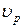Showing posts with label Waves and Oscillation. Show all posts
Showing posts with label Waves and Oscillation. Show all posts

## Phase velocity

Phase velocity is the velocity with which a plane progressive wave front travels forward. Phase velocity is symbolized by. It has a constant phase =## Group velocity

The group velocity () is the speed with which the point of reinforcement of the resultant wave travels. The group velocity is different from the individual wave velocity.

## Phase angle

Phase angle is defines as the fraction of the time interval that has lapsed since the particle has crossed the mean position of rest in the positive direction. It is also equal to the angle swept by the radius vector since the vibrating particle last crossed its mean position of rest.

### Stationary waves:

When two simple harmonic waves of the same amplitude, frequency and time period travel in opposite directions in a straight line, the resultant wave obtained is called a stationary or a standing wave. The formation of stationary waves is due to the superposition of the two waves on the particles of the medium.

### Transverse waves:

In transverse wave motion, the particles of the medium vibrate at right angles to the direction of propagation of wave. Example: Light waves are transverse waves.

### Longitudinal wave motion:

In longitudinal wave motion, particles of the medium vibrate along the direction of propagation of the wave. Example: Sound waves are longitudinal waves.

### Wave motion:

Wave motion is a form of disturbance produced in the medium by the repeated periodic motion of the particles of the medium. Here, only the waves travel forward whereas the particles of the medium vibrate about their mean positions.

### Resonance:

When the forced frequency is equal to the natural frequency of vibration of the body, resonance takes place. Resonance is a vibration of large amplitude produced by a relatively small vibration near the same frequency of vibrations as the natural frequency of the resonating system.

### Undamped free vibrations:

When a bob of a simple pendulum (in vacuum) is displaced from its mean position and left, it executes simple harmonic motions. The pendulum will continue to oscillate with the same time period and amplitude for any length of time. In such cases there is no loss of energy by friction or otherwise. In all similar cases, the vibrations will be undamped free vibrations.

### Lissajous’ figure:

When a particle is influenced simultaneously by two simple harmonic motions at right angles to each other, the resultant motion of the particle traces a curve. These curves are called Lissajous’ figure.

### Simple harmonic motion:

The type of motion where the acceleration is directed towards a fixed point (the mean position of rest) and is proportional to the displacement of the vibrating particle is called simple harmonic motion.